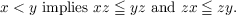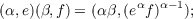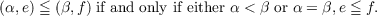#### Vol. 15, No. 2, 1965

 Download this articleFor screen For printingRecent Issues Vol. 325: 1  2 Vol. 324: 1  2 Vol. 323: 1  2 Vol. 322: 1  2 Vol. 321: 1  2 Vol. 320: 1  2 Vol. 319: 1  2 Vol. 318: 1  2Online Archive Volume: Issue:The Journal Subscriptions Editorial Board Officers Contacts Submission Guidelines Submission Form Policies for Authors ISSN: 1945-5844 (e-only) ISSN: 0030-8730 (print) Special Issues Author Index To Appear Other MSP Journals
Proper ordered inverse semigroups

### Tôru Saitô

Vol. 15 (1965), No. 2, 649–666
##### Abstract

Let S be an ordered inverse semigroup, that is, an inverse semigroup with a simple order < which satisfies the condition:Let E be the subsemigroup of S constituted by all the idempotents of S. By a result of Munn, Γ = S∕σ is an ordered group, where σ is the congruence relation such that xσy if and only if ex = ey for some e E. An ordered inverse semigroup S is called proper if the σ-class I which is the identity element of Γ contains only idempotents of S.

In a proper ordered inverse semigroup S, let Γ(e)(e E) be the set of those members of Γ which intersect nontrivially with Re. Each element of S can be represented in the form (α,e), where e E and α Γ(e). We define eα = a1a E, where a = (α,e). Then Γ(e) and eα satisfy the following six conditions:

(i) eEΓ(e) = Γ;

(ii) I Γ(e) and eI = e;

(iii) if f e in the semilattice with respect to the natural ordering of the commutative idempotent semigroup E and α Γ(e), then α Γ(f) and fα eα in the semilattice E;

(iv) if α Γ(e) and β Γ(eα), then αβ Γ(e) and eαβ = (eα)β;

(v) if α Γ(e), then α1 Γ(eα);

(vi) if α Γ(e) Γ(f) and e f, then eα fα.

Also the product and the order in S determined byNext we prove conversely a theorem asserting that, for an ordered commutative idempotent semigroup E and an ordered group Γ, if Γ(e) and eα satisfy the six conditions above, then the set {(α,e);e E,α Γ(e)} is a proper ordered inverse semigroup with respect to the product and the order mentioned above. Besides this, we present other characterizations of special cases.

Primary: 06.70
Secondary: 20.00
##### Milestones